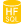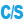WINDEVWEBDEVWINDEV MobileOthers.StatCalculate (Function)In french: .StatCalculeAvailable only with these kinds of connection Performs various statistical calculations on the file keys. This function is equivalent to HAccelerateSpeed.These statistics are used when implementing a filter, creating a query or creating a view on the data file. The HFSQL engine analyzes the selection condition, then uses these statistics to define the most discriminating items that will be used to optimize iterations on data files.The statistics are used to get information about the content of the data file when they are calculated. Therefore, the more modifications are performed in the file after this calculation, the less these statistics represent the true content of the data file. The more records the data file contains, the less the modification of a single record impacts the overall statistics.Caution: .StatCalculate prevents from writing to the data file during the calculation of statistics (an iteration is performed for each key in the data file).The statistics can be calculated in background task without locking the data file in write mode. Remark: In order for the statistical calculation to be efficient, this calculation must be performed on all data files (for a multi-file query for example).Example// Statistics on the CustName key of the Customer data fileCustomer.StatCalculate(CustName)Syntax = .StatCalculate([ [,